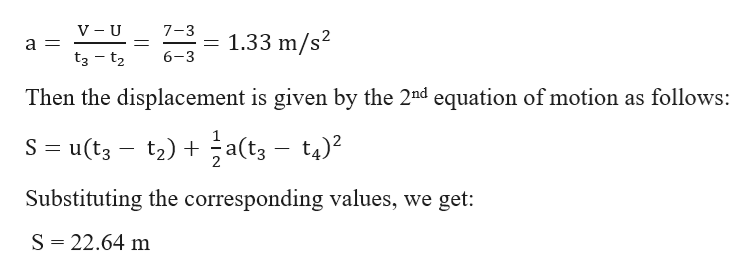# Physics Question

Question
check_circleExpert Solution
Step 1

Part A:

From the given graph we can see that the velocity of the body remains constant from

t = 0 s to t = 3 s which means the acceleration is Zero.

Step 2

From the given graph we can see that the velocity (V) of the is 3 m/s from t1 = 0 s to t2 = 3 s

Hence the displacement (S) is given by:

S = V × (t2 – t1)

S = 3 × 3

S = 9 m

Step 3

From the given graph we can see that the velocity of the body at t2 = 3 s is, initial velocity (U), 3 m/s. ...help_outlineImage TranscriptioncloseV U 7-3 1.33 m/s2 a = 6-3 t3 t2 Then the displacement is given by the 2nd equation of motion as follows: =u(t3- t2)a(t3 - t4)2 Substituting the corresponding values, we get: S 22.64 m fullscreen

### Want to see the full answer?

See Solution

#### Want to see this answer and more?

Solutions are written by subject experts who are available 24/7. Questions are typically answered within 1 hour*

See Solution
*Response times may vary by subject and question
Tagged in

### Physics